# The MI Procedure

### Combining Inferences from Multiply Imputed Data Sets

With m imputations, m different sets of the point and variance estimates for a parameter Q can be computed. Suppose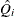and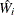are the point and variance estimates from the ith imputed data set, i = 1, 2, …, m. Then the combined point estimate for Q from multiple imputation is the average of the m complete-data estimates: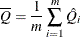Suppose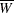is the within-imputation variance, which is the average of the m complete-data estimates,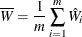and B is the between-imputation variance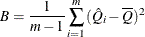Then the variance estimate associated with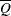is the total variance (Rubin, 1987)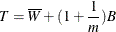The statistic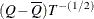is approximately distributed as t with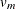degrees of freedom (Rubin, 1987), where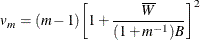The degrees of freedomdepend on m and the ratio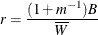The ratio r is called the relative increase in variance due to nonresponse (Rubin, 1987). When there is no missing information about Q, the values of r and B are both zero. With a large value of m or a small value of r, the degrees of freedomwill be large and the distribution ofwill be approximately normal.

Another useful statistic is the fraction of missing information about Q: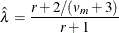Both statistics r andare helpful diagnostics for assessing how the missing data contribute to the uncertainty about Q.

When the complete-data degrees of freedom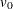are small, and there is only a modest proportion of missing data, the computed degrees of freedom,, can be much larger than, which is inappropriate. For example, with m = 5 and r = 10%, the computed degrees of freedom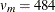, which is inappropriate for data sets with complete-data degrees of freedom less than 484.

Barnard and Rubin (1999) recommend the use of adjusted degrees of freedom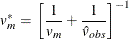where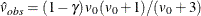and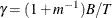.

Note that the MI procedure uses the adjusted degrees of freedom,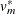, for inference.